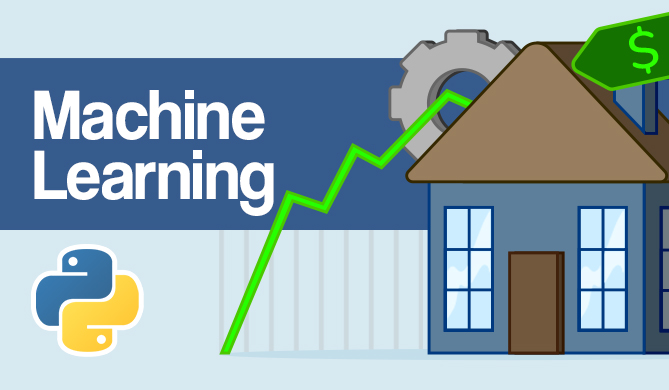RandomizedSearchCV with XGBoost in Scikit-Learn Pipeline

# RandomizedSearchCV with XGBoost in Scikit-Learn PipelineRandomizedSearchCV and GridSearchCV allow you to perform hyperparameter tuning with Scikit-Learn, where the former searches randomly through some configurations (dictated by n_iter) while the latter searches through all of them.

XGBoost is an increasingly dominant library, whose regressors and classifiers are doing wonders over more traditional implementations, and is based on an extreme version of gradient boosting.

It plays well with Scikit-Learn and its models can in most cases be used in place of Scikit-Learn models.

In this Byte - you'll find an end-to-end example of a Scikit-Learn pipeline to scale data, fit an XGBoost's XGBRegressor and then perform hyperparameter tuning with Scikit-Learn's RandomizedSearchCV.

First, let's create a baseline performance from a pipeline:

import sklearn
from sklearn import datasets
from sklearn.model_selection import train_test_split
from sklearn.preprocessing import MinMaxScaler
from sklearn.pipeline import Pipeline

from xgboost import XGBRegressor

X, y = datasets.fetch_california_housing(return_X_y=True)
X_train, X_test, y_train, y_test = train_test_split(X, y)

pipeline = Pipeline([('scaler', MinMaxScaler()), ('regressor', XGBRegressor())])
pipeline.fit(X_train, y_train)


Let's score it:

pipeline.score(X_test, y_test)
# 0.7752702631435812


Awesome, an R2 score of 0.77! Let's perform hyperparameter tuning on the pipeline. You can access each element of the pipeline to set their properties by using their name in the pipeline with __. If you're unsure of the names, you can always get all of the configurable params as:

pipeline.get_params().keys()
# dict_keys([..., 'scaler__copy', 'scaler__feature_range', 'regressor__base_score', 'regressor__booster', 'regressor__colsample_bylevel', ...


Let's make a hyperparameter grid and initialize the search:

## Get free courses, guided projects, and more

hyperparameter_grid = {
'regressor__n_estimators': [100, 500, 1000, 2000],
'regressor__max_depth': [3, 6, 9, 12],
'regressor__learning_rate': [0.01, 0.03, 0.05, 0.1]
}

random_cv = sklearn.model_selection.RandomizedSearchCV(estimator=pipeline,
param_distributions=hyperparameter_grid,
cv=3,
n_iter=5,
scoring = 'neg_root_mean_squared_error',
n_jobs = -1,
verbose = 5,
return_train_score = True,
random_state=42)

random_cv.fit(X_train, y_train)


The search will take a bit of time, and results in something along the lines of:

Fitting 3 folds for each of 5 candidates, totalling 15 fits

RandomizedSearchCV(cv=3,
estimator=Pipeline(steps=[('scaler', MinMaxScaler()),
('regressor', XGBRegressor())]),
n_iter=5, n_jobs=-1,
param_distributions={'regressor__learning_rate': [0.01, 0.03,
0.05,
0.1],
'regressor__max_depth': [3, 6, 9, 12],
'regressor__n_estimators': [100, 500,
1000,
2000]},
random_state=42, return_train_score=True,
scoring='neg_root_mean_squared_error', verbose=5)


best_pipe = random_cv.best_estimator_


Or inspect it:

best_pipe
# Pipeline(steps=[('scaler', MinMaxScaler()),
#                ('regressor', XGBRegressor(max_depth=9, n_estimators=1000))])


Finally, let's score it for reference:

best_pipe.score(X_test, y_test)
# 0.8309779703673673


Great, we've increased the score from 0.77 to 0.83 through a simple, short search!Note: Don't score() the random_cv object, but rather, the best found pipeline.

Last Updated: July 1st, 2022

### You might also like...

David LandupAuthor

Entrepreneur, Software and Machine Learning Engineer, with a deep fascination towards the application of Computation and Deep Learning in Life Sciences (Bioinformatics, Drug Discovery, Genomics), Neuroscience (Computational Neuroscience), robotics and BCIs.

Great passion for accessible education and promotion of reason, science, humanism, and progress.Project

### Real-Time Road Sign Detection with YOLOv5

# python# machine learning# computer vision# pytorch

If you drive - there's a chance you enjoy cruising down the road. A responsible driver pays attention to the road signs, and adjusts their...David Landup
DetailsProject

### Hands-On House Price Prediction - Machine Learning in Python

# python# machine learning# scikit-learn# tensorflow

If you've gone through the experience of moving to a new house or apartment - you probably remember the stressful experience of choosing a property,...Details